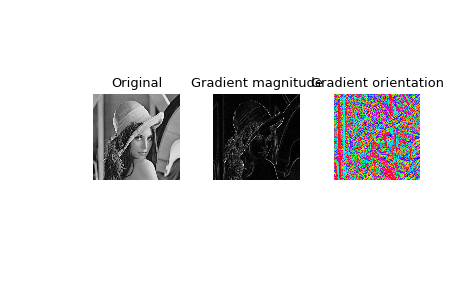# scipy.signal.convolve2d¶

scipy.signal.convolve2d(in1, in2, mode='full', boundary='fill', fillvalue=0)[source]

Convolve two 2-dimensional arrays.

Convolve in1 and in2 with output size determined by mode, and boundary conditions determined by boundary and fillvalue.

Parameters: in1, in2 : array_like Two-dimensional input arrays to be convolved. mode : str {‘full’, ‘valid’, ‘same’}, optional A string indicating the size of the output: full The output is the full discrete linear convolution of the inputs. (Default) valid The output consists only of those elements that do not rely on the zero-padding. same The output is the same size as in1, centered with respect to the ‘full’ output. boundary : str {‘fill’, ‘wrap’, ‘symm’}, optional A flag indicating how to handle boundaries: fill pad input arrays with fillvalue. (default) wrap circular boundary conditions. symm symmetrical boundary conditions. fillvalue : scalar, optional Value to fill pad input arrays with. Default is 0. out : ndarray A 2-dimensional array containing a subset of the discrete linear convolution of in1 with in2.

Examples

Compute the gradient of an image by 2D convolution with a complex Scharr operator. (Horizontal operator is real, vertical is imaginary.) Use symmetric boundary condition to avoid creating edges at the image boundaries.

>>> from scipy import signal
>>> from scipy import misc
>>> lena = misc.lena()
>>> scharr = np.array([[ -3-3j, 0-10j,  +3 -3j],
...                    [-10+0j, 0+ 0j, +10 +0j],
...                    [ -3+3j, 0+10j,  +3 +3j]]) # Gx + j*Gy
>>> grad = signal.convolve2d(lena, scharr, boundary='symm', mode='same')

>>> import matplotlib.pyplot as plt
>>> fig, (ax_orig, ax_mag, ax_ang) = plt.subplots(1, 3)
>>> ax_orig.imshow(lena, cmap='gray')
>>> ax_orig.set_title('Original')
>>> ax_orig.set_axis_off()
>>> ax_mag.set_axis_off()
>>> ax_ang.imshow(np.angle(grad), cmap='hsv') # hsv is cyclic, like angles